Courses

# Alternating Current and its Average and RMS Value Class 12 Notes | EduRev

## Class 12 : Alternating Current and its Average and RMS Value Class 12 Notes | EduRev

The document Alternating Current and its Average and RMS Value Class 12 Notes | EduRev is a part of the Class 12 Course Physics For JEE.
All you need of Class 12 at this link: Class 12

Alternating Current:

1. Alternating Current:

Until now, we have studied only circuits with direct current (dc) which flows only in one direction. The primary source of emf in such circuit is a battery. When a resistance is connected across the terminals of the battery, a current is established in the circuits, which flows in a unique direction from the positive terminal to the negative terminal via the external resistance.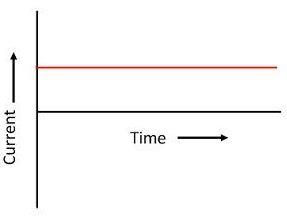Fig: Direct current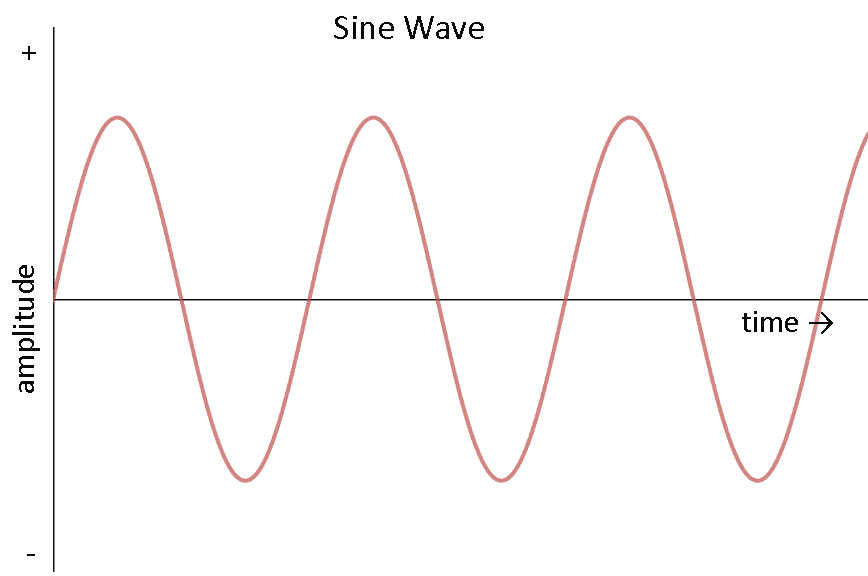Fig: Alternating current

But most of the electric power generated and used in the world is in the form of alternating current (ac), the magnitude of which changes continuously with time and direction is reversed periodically (as shown in figure III & IV) and it is given by

i = i0 sin (ωt + φ)

Here i is instantaneous value of current i.e., the magnitude of current at any instant of time and i0 is the maximum value of current which is called peak current or the current amplitude and the current repeats its value after each time interval T =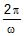as shown in figure. This time interval is called the time period and w is angular frequency which is equal to 2π times of frequency f.

ω = 2πfFig: AC wave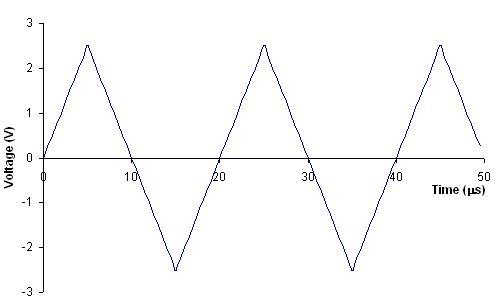Fig: Triangular wave

The current is positive for half the time period and negative for remaining half period. It means that the direction of current is reversed after each half time period. The frequency of ac in India is 50 Hz.

An alternating voltage is given by:

V = V0 sin (ωt + φ)

It also varies alternatively as shown in the figure (b), where V is instantaneous voltage and V0 is peak voltage. It is produced by ac generator also called as ac dynamo.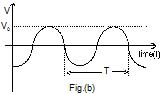AC Circuit: An ac circuit consists of circuit element i.e., resistor, capacitor, inductor or any combination of these and a generator that provides the alternating current as shown in figure. The ac source is represented by symbol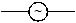in the circuit.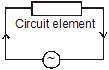2. AVERAGE AND RMS VALUE OF ALTERNATING CURRENT:

2.1 Average current (Mean current):

As we know an alternating current is given by

i = i0 sin (ωt + f) ...(1)

The mean or the average value of ac over any time T is given by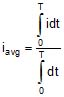Using equation (1)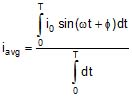In one complete cycle, the average current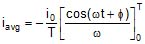= -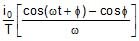=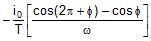= 0 (as ωT = 2π)

Since ac is positive during the first half cycle and negative during the other half cycle so iavg will be zero for long time also. Hence the dc instrument will indicate zero deflection when connected to a branch carrying ac current. So it is defined for either positive half cycle or negative half cycle.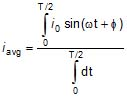=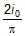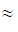0.637 i0

Similarly Vavg =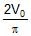0.637 V0

Ex. 1 If a direct current of value a ampere is superimposed on an alternating current i = b sin wt flowing through a wire, what is the effective value of the resulting current in the circuit ?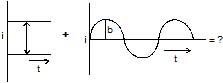Ans: As current at any instant in the circuit will be,

i = idc + iaca + b sin ωt

So, ieff =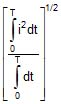=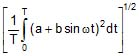i.e., =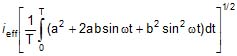but as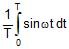= 0 and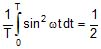So, ieff =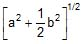2.2 R.M.S Value of alternating current:

The notation rms refers to root mean square, which is given by square root of mean of square current.

i.e.,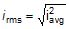i2avg =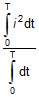=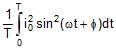=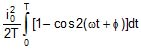=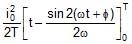=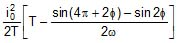=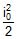irms =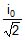» 0.707 i0

Similarly the rms voltage is given by

Vrms =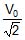» 0.707 V0

The significance of rms current and rms voltage may be shown by considering a resistance R carrying a current i = i0 sin (wt + f)

The voltage across the resistor will be

VR = Ri = (i0R) sin (ωt + φ)

The thermal energy developed in the resistor during the time t to t + dt is

i2 R dt = i02R sin2(ωt + φ) dt

The thermal energy developed in one time period is

U =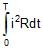=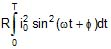= RT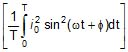= i2rms RT

It means the root mean square value of ac is that value of steady current, which would generated the same amount of heat in a given resistance in a given time.

So in ac circuits, current and ac voltage are measured in terms of their rms values. Likes when we say that the house hold supply is 220 V ac it means the rms value is 220 V and peak value is = 311 V.

Ex. 2 If the voltage in an ac circuit is represented by the equation, V = sin (314t - φ),calculate (a) peak and rms value of the voltage, (b) average voltage, (c) frequency of ac.

Ans: (a) For ac voltage,

V = V0 sin (ωt - φ)

The peak value of voltage

V0 =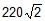= 311 V

The rms value of voltage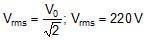(b) Average voltage in full cycle is zero. Average voltage in half cycle is

Vavg =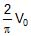=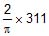= 198.17 V

(c) As ω = 2πf, 2ωf = 314

i.e., f =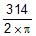= 50 Hz

Ex. 3 The electric current in a circuit is given by i = i0 (t/T) for some time. Calculate the rms current for the period t = 0 to t = T.

Ans: The mean square current is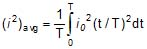=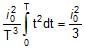Thus, the rms current is

irms =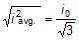Offer running on EduRev: Apply code STAYHOME200 to get INR 200 off on our premium plan EduRev Infinity!

## Physics For JEE

187 videos|516 docs|263 tests

,

,

,

,

,

,

,

,

,

,

,

,

,

,

,

,

,

,

,

,

,

;